# Science of Uniaxial Deformation

Uniaxial Strain and Test ConditionsUniaxial Deformation simply means that you push or pull in one direction only.Here are two examples of uniaxial deformation occurring in daily life: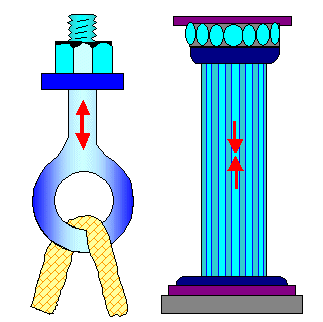Uniaxial deformation Here we pull, producing tensile stress and strain. Here we push, producing compressive stress and strain.When we do a tensile test we elongate the specimen at a defined strain rate by applying the right stress via a feed-back loop.
We need a few definitions now:
• s = stress = force per area. The dimension of stress is [s] = Nm–2. 1 Newton per square meter is called 1 Pa (Pascal)
• e = strain = (l(s) – l0)/l0. l(s) is the length of the specimen at the applied stress s; l0 is the initial length or l0 = l(s = 0). Strain is an entity without a unit!
Strain as defined here (in contrast to the main text) times 100 gives the elongation in percent (%). Note that "percent" is not a unit, just a relation.
• de/dt, the derivative of the strain with respect to time t, is the strain rate.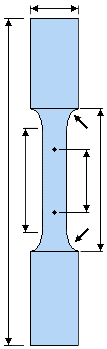Standard tensile specimenFor tensile testing you need a standardized specimen (see figure on the right; all arrows indicate well-defined dimensions) with a size that fits your machine. Since the maximum stress the machine can apply is limited, your specimen should not be too big - the machine then can't break it.
You also need to measure the strain and the stress with some kind of precision. There are many ways for doing that but I will not go into this.
The settings for the strain rate are limited too, of course. For very low strain rates you would have to wait very long until your specimen finally fractures; they are also difficult to measure. For very large strain rates you might need more stress than the machine can muster.
Of course, you need to measure the strain all the time and feed the results into some electronic feed-back loop that controls the stress to the amount needed to produce the desired strain rate.Temperature control is also not easy. You could put the whole machine in a room with defined temperature but that is not too practical if you deviate too much from room temperature. This leaves only to construct a "temperature chamber" around the specimen, not an easy task but doable.
OK—after you came up with a few 100.000 Dollars for buying a machine, found a suitable room and a machine shop that can shape the specimen, you may now commence with uniaxial testing of materials.

Tensile Test – DefinitionsWhen we do a tensile test we will get a stress-strain diagram as a result. Below is one (from some steel) as it comes out of the machine. My machine speaks German so I added the translations in red.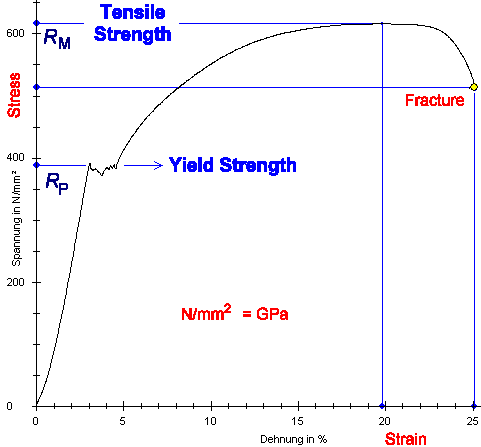Tensile stress - strain diagram of some steelThe machine indicates the stress and strain values for the (ultimate) tensile strength and fracture by blue lines. It can do that because it is smart enough to find the maximum of a curve and the end of one.
It does not indicate the critical yield strength RP because it is not smart enough to detect that. In fact, there are special definitions and recipes to arrive at a number for RP but I will not go into that.
In the curve shown the location of RP is plain to see but that is not always so. We also see that some strange things go on for a while after RP was reached. The curve gets kind of wobbly, and much is made of this in real testing.
It is not unusual that the stress - strain curve wiggles a bit or runs through a small peak as soon as plastic deformation begins at RP. That just signifies that before plastic deformation can proceed in a major way by dislocation movement, the dislocations either have to be generated first, or ripped off from the obstacles that pinned them down, or both. That happens at somewhat different stresses in different parts of the sample, and that's why the curve might become "noisy". It's an important phenomena and a nuisance. Since at this point we do not yet know what dislocations are, I will get back to this point much later.Of course, the slope of the stress s-strain e curve in the elastic region gives Youngs' modulus in a straightforward way as s/e or better, since we don't have a perfect straight line, as the slope or
Y = ds/deA maximum in any curve is a useful thing because it is easily detected and interpreted. In a stress-strain curve it denotes the ultimate tensile strength RM because, as the word implies, stress above RM would invariably produce fracture. RM of course, is a useful property to know for engineering materials.Unfortunately, in the science of materials, RM is rather unimportant. It denotes a stress that does not truly exist in the material. It is easy to see why.
The tensile test machine calculates the stress by dividing the force it applies by the initial cross-sectional area of the specimen. That is fine for the beginning of the experiment, when the actual cross-sectional area of the specimen is close to its initial one. However, as the specimen is stretched well beyond the yield point, it must get thinner because its volume cannot change a lot. The cross-section thus decreases and the true stress would be: applied force divided by the actual and smaller cross-sectional area.It is, of course, quite easy to calculate the true stress s* (that's what we really call it). It is always larger than the nominal stress s that the machine uses. Going through the numbers (assuming no volume change) we obtain

s*  =   s · (e + 1)Alas! If we replot the stress-strain curve now for true stress, the useful maximum is gone! That's one reason why we usually don't do that. The other reason is that in any application the trues stress is irrelevant. You want to know when failure occurs relative to the dimensions of the material you used, not the dimensions it might assume.Things get worse. From a scientific point of view, the nominal strain we used is also not the real thing. We need the true strain.Now why is that? Imagine you strain your specimen just by 1 %. Then you strain it again by 1 %, and again, and again. After you did that 10 times, how large is the total strain?
If you go for 10 × 1 % = 10 %, you are probably not a rich person. If you get 10 % interest on your capital of 100 \$, how much accumulated interest do you have after 5 years? 5 × 10 % = 50 %?
No! After the first year you get 10 \$ interest and your capital is now 110 \$. After the second year, you get 11 \$ interest on that. Your total capital after 5 years is \$ (100 + 10 + 11 + 12 + 13 + 14) = \$ 160 and your accumulated interest is 60 \$ and not 50 \$.
It's called compound interest calculation and if you ever learned about that in school, you hated it.We need to do the same thing for the strain if we want to consider the true strain e* as being the final strain after straining bit by bit. Of course, it is again (almost) easy to calculate, here is the result.

e*  =   ln (e + 1)Sorry. The dreaded logarithms raise their ugly heads! Even the logarithm naturalis to base e!
Either you know what that means or you don't. Consulting this module might refresh your memory but will not substitute for all the (still rather elementary) math you may have been deprived off.While we usually do not plot stress-strain diagrams with true strain either, we need it as soon as we calculate properties from a stress-strain curve like the fracture energy. Or as soon as we start comparing tensile and compressive testing, see below.There is one more thing that we can learn form uniaxial testing: Above I stated that the specimen gets thinner because its volume cannot change a lot. That is certainly true but the question we ask now is "how thin?"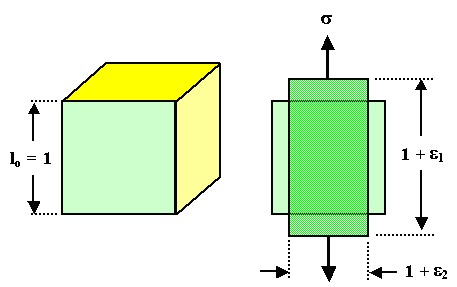Specimen contractions perpendicular to the pull axis.Well, we don't know so we must measure it. We have some strain e2 perpendicular to the pull direction and thus a length change of 1 + e2 in the geometry shown above. Since the sample gets longer but thinner, e2 must be negative. We have whar is called "lateral contraction".
Measuring e1 and e2 allows to define a new material parameter, called Poisson's ratio n

n  =  – e1
e2You should have a question now: "why don't we just calculate n once and for all, assuming that the volume stays constant". The answer is that we do that, of course. The result is
n(const. volume) = 0.5. Fine. Now look at the measured values of Poisson's ratio:

Material   Poisson's ratio n
Polymers   0.3 - 0.4
Metals   0.25 - 0.35
Steel
Aluminum
Magnesium
0.3
0.33
0.35
Diamond 0.2 (smallest value)
Const. volume   0.5The volume of a specimen in a tensile test, obviously, does not stay constant. No big deal. Why should it? After all, the distance between the atoms gets considerably larger when you pull at them.
We have no choice but to live with measured numbers for Poisson's ratio. Yes, it is never far from 0.5 and thus is not as important as Young's modulus. It is nevertheless needed for any calculations that try to do better than to just give orders of magnitude.

Tensile Test: Ultimate Tensile Strength and NeckingTaking stress-strain curves with nominal stress and strain produces the maximum discussed above, conveniently denoting the ultimate tensile strength RM. It also denotes necking, the phenomenon that the specimen starts to develop a neck, a region where it is thinner than in the rest of its body. In real life necked (and ruptured) specimen look as shown in this link; schematically it looks like this: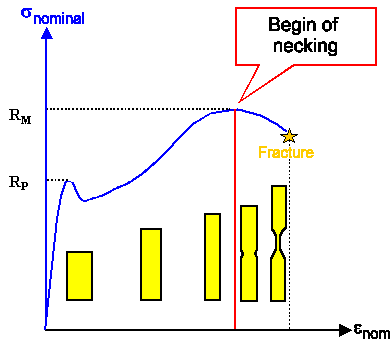Necking and RMWhy is the maximum of the (nominal) stress-strain curve and the beginning of necking related?The answer is found as soon as we look at the general dependence of most stress-strain curves on the strain rate de/dt. While curves taken at different strain rates may not be very different, you always need somewhat larger stresses at higher strain rates to achieve the same strain.
Keeping this in mind we now look at a specimen under tensile stress and assume that in a small part (region 2 in the figure below) the strain is a little bit larger than in the rest or region 1. Maybe there is a little defect, whatever. The question is: what is going to happen in this case?The answer is found in the figure below. It shows two stress-strain curves for two different stress rates de/dt; in the figure abbreviated by the usual dot on top of the function (not possible in HTML). The exact shape of the two curves doesn't matter; the only important point is that it always takes more stress to produce the same strain a higher strain rates.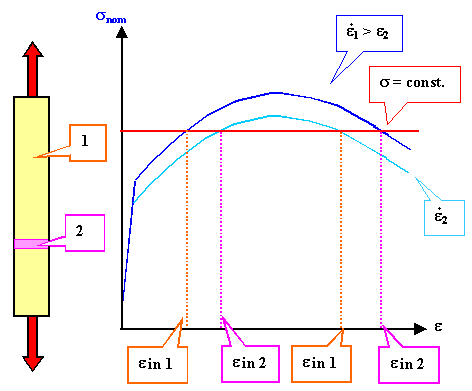Since the nominal stress is same everywhere, region 2 with the larger strain will be found:
• On the lower curve to the left of the maximum.
• On the upper curve to the right of the maximum.To the left of the maximum region 1 thus will experience a larger strain rate than region 2 so it will always catch up with region 1 again, and the two regions equilibrate again.
To the right of the maximum, region 2 experiences the larger strain rate and strains faster and faster in comparison to region 1. Region 2 thus becomes thinner and thinner since the volume must be (approximately) preserved. The true stress becomes larger and larger since the cross-sectional area becomes smaller. Since the total strain rate must be unchanged, straining occurs more and more only in the necking region. The whole system becomes unstable and runs off to fracture.

Compressive TestingIn compressive testing something new might occur that we have to deal with first: The whole sample bends outwards while still in the elastic region. Its length in the bend state is pretty much what it was without stress but since it is bend, the distance between the two ends is smaller.
We are no longer doing uniaxial testing now! This is shown schematically in the figure below.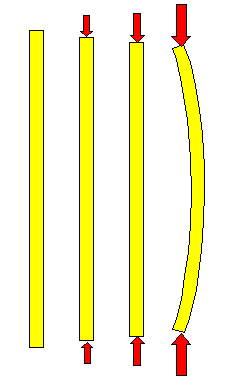Outward bending occurs as soon as some critical compressive stress is reachedYou know that, of course. It may happen when you try to stab something with your sword. It is clear without doing calculations that bending happens more easily for slender specimen than for squat ones. Calculations, which are not very difficult to do, give clear criteria for bending under compression.For uniaxial testing, however, bending under compression is irrelevant because if it occurs you have no longer uniaxial testing. Your specimen now experiences complex stresses. On the convex side is under tensile stress, on the concave side it is under compressive stress, and in the middle there is no stress at all. We will need that later, so it is a good idea to look a the following figure and ponder its meaning now: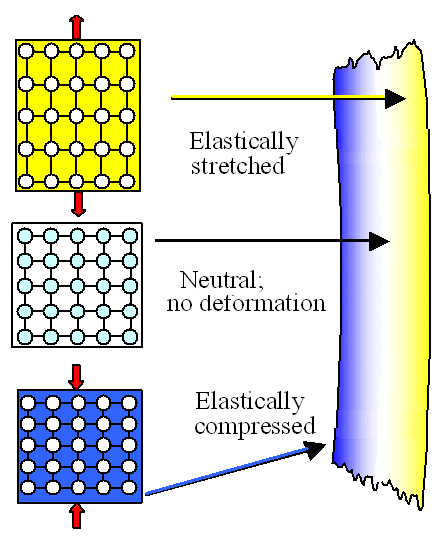Bend specimen experience tensile and compressive stressIf our specimen bows out as in the figure above, the outer (right) part will be under tensile stress since it is longer than the original length.
By the same token, the inside part will be under compressive stress; it is now shorter.
It follows that there must be a neutral part somewhere in the interior with no stress or strain, since its length has not changed. In the example above, we can expect that the neutral part is right in the middle.Bow like that cannot occur under tensile testing, and that is one of the reason why we prefer the tensile test. If we need to do compressive testing, we need to make our specimen short and thick to avoid "bow". If we do that and now go for both modes, i.e. push and pull we get stress-strain curves as shown below.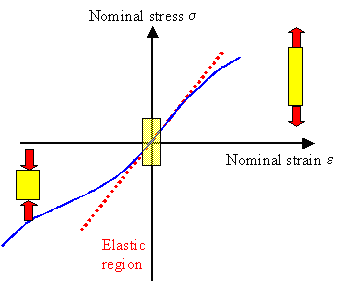Stress - strain curves for tensile and and compressive testing are quite different for nominal stress and strain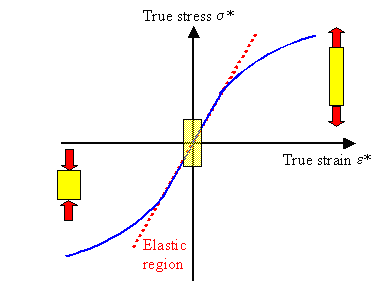Stress - strain curves for tensile and and compressive testing are almost identical for for true stress and strainGoing from nominal stress and strain to true stress and strain simply means that you take into account that the cross-sectional areas change during deformation. If you elongate a specimen it gets thinner, if you compress it, it gets thicker. Stress is force divided by area and we need to take that into account of we want to be precise. Then everything is fine - except that calculating true stress and strain is surprisingly difficult to do! So people tend to stay with the nominal stress and strain , knowing that they could always convert it if needed.So I did not cheat you when I claimed that "the stress strain-curve for not too large stresses (in compressive testing) looks the same, just inverted, as in tensile testing. Well, yes - but only if you avoid bow and take a little care to adjust for the changed geometry.Adjusting the geometry is what we do when we moved to true stress and strain. If the effort for doing this is seen as inconsequential or overbearing depends on one's perspective.So there is more to uniaxial testing then meets the eye. Yes, but don't deceive yourself. I only scratched the surface here. There is far more to that simple experiment then I could relate in this short "science" module.Galileo Galilei3.1.2 Stiff or Hard?Fracture Mechanics IMyths and Bullshit Around Quenching3.1.4 A Bit More About Tensile TestingSpring Model and Properties of CrystalsInhomogeneous DeformationPolishing CrystalsBandingComposite MaterialsBending of Beams3.1.1 Breaking Things in StyleExponentials and LogarithmsCrystal ModelsRiveting, Soldering, Liquid Welding Plus Gluing and ScrewingSteel PropertiesDisplacement and StrainThermal StressScience of DeformationFracture Mechanics II12.2.2 Sword Bending: Deformation and Fracture12.2.3 Sword Buckling

© H. Föll (Iron, Steel and Swords script)